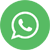DATA SCIENCE-Data Mining -Unsupervised Learning

# Data Mining

Is also known as “Machine Learning”
Data Mining is divided into two subcategories
1. Unsupervised Learning
2. Supervised Learning

Unsupervised Technique:
If Output(Y) is not Known, then we will go for Unsupervised Technique.
A Few of Unsupervised Data Mining Techniques are:
• Association Rules
• Recommendation system
• Clustering
• Dimension Reduction Techniques
• Network Analysis

Association Rules: –
Association Rules are also known as Market Basket Analysis & Affinity Analysis

“IF” part = Antecedent = A
“THEN” part = Consequent = C

Apriori Algorithm:

? Set minimum support criteria
? Generate list of one-item sets that meet the support criterion
? Use list of one-item sets to generate list of two-item sets that meet support criterion
? Use list of two-item sets to generate list of three-item sets that meet support criterion
? Continue up through k-item sets

a. Support:
? Consider only combinations that occur with higher frequency in the database
? Support is the criterion based on frequency

Formula:

Percentage / Number of transactions in which IF/Antecedent & THEN / Consequent appear in the data

Mathematically:
# transactions in which A & C appear together / # Total no. of transactions

b. Confidence
Formula: Percentage of If/Antecedent transactions that also have the Then/Consequent item set

Mathematically:

P (Consequent | Antecedent) = P (C & A) / P(A)

# transactions in which A & C appear together / # transactions with A

Confidence – Weakness
If antecedent and consequent have:
High Support => High / Biased Confidence
c. Lift Ratio:

Confidence / Benchmark confidence

Benchmark assumes independence between antecedent & consequent:

Benchmark confidence:

P(C|A) = P (C & A) / P(A) = P(C) X P(A) /P(A) = P(C)

# transactions with consequent item sets / # transactions in database

Interpreting Lift:
Lift > 1 indicates a rule that is useful in finding consequent item sets